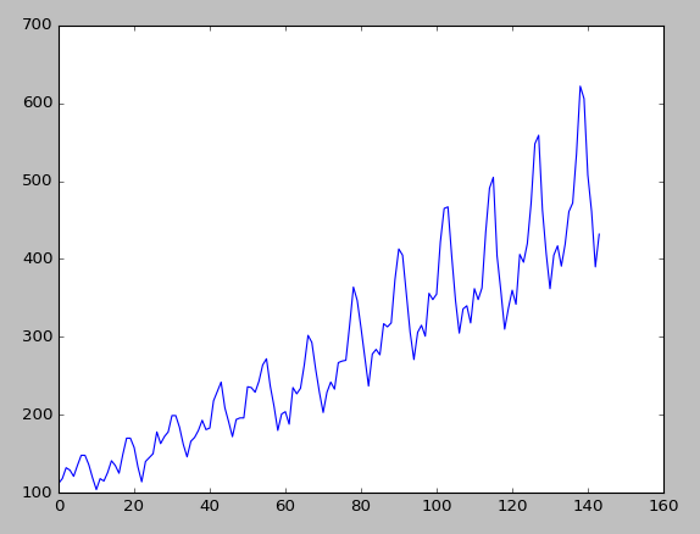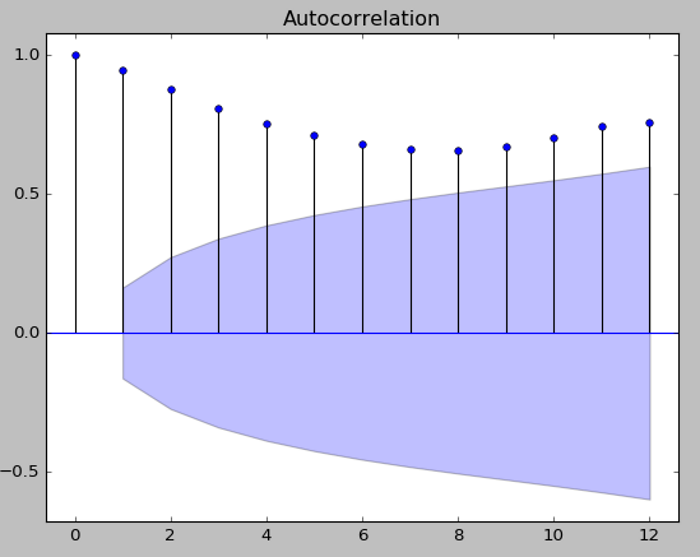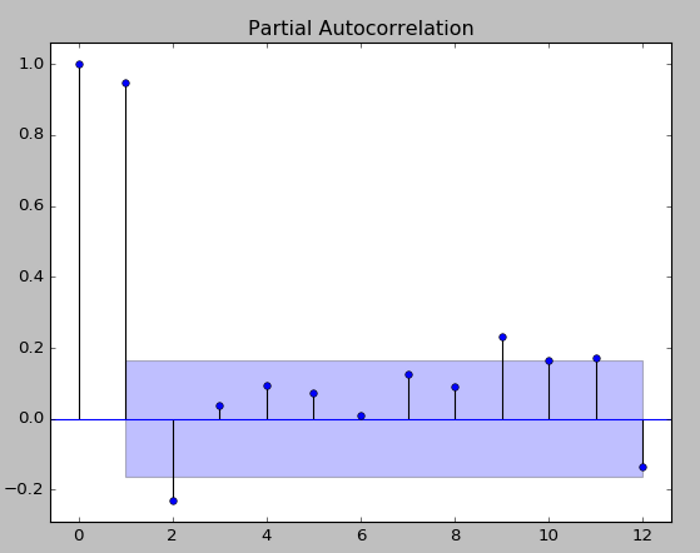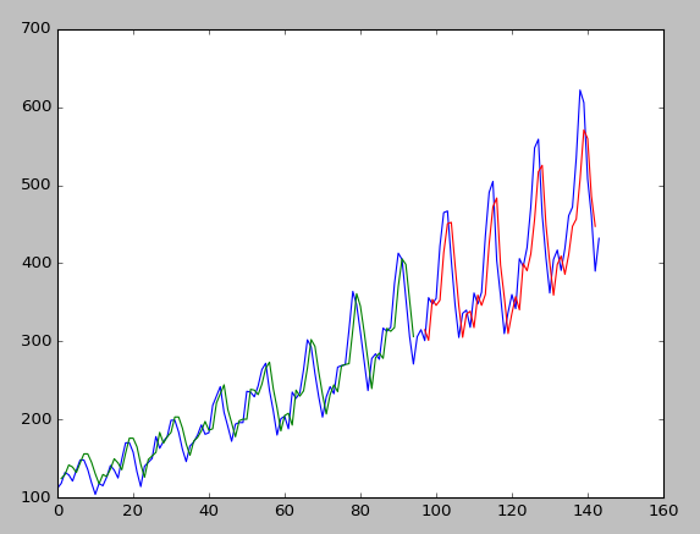#2018 iT 邦幫忙鐵人賽DAY 23
3
AI & Machine Learning

## Neural Network 作法

``````import pandas
import matplotlib.pyplot as plt
dataset = pandas.read_csv('international-airline-passengers.csv', usecols=, engine='python', skipfooter=3)
plt.plot(dataset)
plt.show()
````````````import numpy as np
from scipy import stats
import pandas as pd
import matplotlib.pyplot as plt
import statsmodels.api as sm
from statsmodels.graphics.api import qqplot
# 畫出 ACF 12 期的效應
sm.graphics.tsa.plot_acf(dataset, lags=12)
plt.show()
# 畫出 PACF 12 期的效應
sm.graphics.tsa.plot_pacf(dataset, lags=12)
plt.show()
``````

** 注意，StatsModels 套件，必須以以下指令安裝，用 pip install 會有錯 **
conda install -c conda-forge statsmodels``````# LSTM for international airline passengers problem with regression framing
import numpy
import matplotlib.pyplot as plt
import math
from keras.models import Sequential
from keras.layers import Dense
from keras.layers import LSTM
from sklearn.preprocessing import MinMaxScaler
from sklearn.metrics import mean_squared_error

# 產生 (X, Y) 資料集, Y 是下一期的乘客數
def create_dataset(dataset, look_back=1):
dataX, dataY = [], []
for i in range(len(dataset)-look_back-1):
a = dataset[i:(i+look_back), 0]
dataX.append(a)
dataY.append(dataset[i + look_back, 0])
return numpy.array(dataX), numpy.array(dataY)

# 載入訓練資料
dataframe = read_csv('international-airline-passengers.csv', usecols=, engine='python', skipfooter=3)
dataset = dataframe.values
dataset = dataset.astype('float32')
# 正規化(normalize) 資料，使資料值介於[0, 1]
scaler = MinMaxScaler(feature_range=(0, 1))
dataset = scaler.fit_transform(dataset)

# 2/3 資料為訓練資料， 1/3 資料為測試資料
train_size = int(len(dataset) * 0.67)
test_size = len(dataset) - train_size
train, test = dataset[0:train_size,:], dataset[train_size:len(dataset),:]

# 產生 (X, Y) 資料集, Y 是下一期的乘客數(reshape into X=t and Y=t+1)
look_back = 1
trainX, trainY = create_dataset(train, look_back)
testX, testY = create_dataset(test, look_back)
# reshape input to be [samples, time steps, features]
trainX = numpy.reshape(trainX, (trainX.shape, 1, trainX.shape))
testX = numpy.reshape(testX, (testX.shape, 1, testX.shape))

# 建立及訓練 LSTM 模型
model = Sequential()
model.fit(trainX, trainY, epochs=100, batch_size=1, verbose=2)

# 預測
trainPredict = model.predict(trainX)
testPredict = model.predict(testX)

# 回復預測資料值為原始數據的規模
trainPredict = scaler.inverse_transform(trainPredict)
trainY = scaler.inverse_transform([trainY])
testPredict = scaler.inverse_transform(testPredict)
testY = scaler.inverse_transform([testY])

# calculate 均方根誤差(root mean squared error)
trainScore = math.sqrt(mean_squared_error(trainY, trainPredict[:,0]))
print('Train Score: %.2f RMSE' % (trainScore))
testScore = math.sqrt(mean_squared_error(testY, testPredict[:,0]))
print('Test Score: %.2f RMSE' % (testScore))

# 畫訓練資料趨勢圖
# shift train predictions for plotting
trainPredictPlot = numpy.empty_like(dataset)
trainPredictPlot[:, :] = numpy.nan
trainPredictPlot[look_back:len(trainPredict)+look_back, :] = trainPredict

# 畫測試資料趨勢圖
# shift test predictions for plotting
testPredictPlot = numpy.empty_like(dataset)
testPredictPlot[:, :] = numpy.nan
testPredictPlot[len(trainPredict)+(look_back*2)+1:len(dataset)-1, :] = testPredict

# 畫原始資料趨勢圖
# plot baseline and predictions
plt.plot(scaler.inverse_transform(dataset))
plt.plot(trainPredictPlot)
plt.plot(testPredictPlot)
plt.show()
``````

## 程式執行

python SimpleLSTM.py## 程式說明

1. 資料檔只有兩欄，日期及乘客數，正規化(normalize) 資料，使資料值介於[0, 1]。
2. 我們要用前期預測當期，故將資料轉為(前期乘客數， 當期乘客數)，當作(X, Y)。
3. 建立及訓練 LSTM 模型，模型很簡單，就只有一個LSTM層及output層。
4. 訓練 LSTM 模型並進行預測。
5. 針對實際值、預測值進行繪圖。

## 結論

### 7 則留言

0

ACF/PACF是顯示當期與落後期數的相關程度，從上圖PACF看，當期(左邊第一條)與上期(左邊第二條)最密切，故使用上一期預測當期。

qq520434 iT邦新手 5 級 ‧ 2019-09-09 16:42:05 檢舉

``````look_back = 1
trainX, trainY = create_dataset(train, look_back)
testX, testY = create_dataset(test, look_back)
``````

qq520434 iT邦新手 5 級 ‧ 2019-09-10 16:15:13 檢舉

skipfooter 是因為最後三列不是資料，故跳掉而已。

qq520434 iT邦新手 5 級 ‧ 2019-09-11 12:29:17 檢舉

1

1. 將最後一筆的乘客數當作X，預測下個月的乘客數，程式如下：
``````# 最後一筆的乘客數當作X，預測下個月的乘客數
last_row = scaler.transform(testY[-1].reshape((-1, 1)))
last_row = numpy.reshape(last_row, (last_row.shape, 1, last_row.shape))
testPredict2 = model.predict(last_row)
#print(testPredict2)
scaler.inverse_transform(testPredict2)
``````
1. 範例是AR(1)的模型，前一期當作X，本期當Y，故有滯後的感覺，可以把look_back = 1 改成 2, 3, ... or n 試試看，再複雜一點，可使用 ARIMA 模型，加上移動平均，會使預測的曲線更平滑，試試看噢。
zehua iT邦新手 5 級 ‧ 2019-11-04 14:16:45 檢舉

LSTM 一次只能預測一期，我發現一個很棒的套件 -- FB Prophet，可一次預測很多期，有興趣可以研究一下。

0

10612201 iT邦新手 5 級 ‧ 2020-06-29 16:20:32 檢舉

0

LSTM是只接受三維數組的資料嗎？
==> 是，請參閱 https://keras.io/api/layers/recurrent_layers/lstm/
inputs: A 3D tensor with shape [batch, timesteps, feature].

time_steps的位置加入1
==> 每一期資料只跟前"一"期有關

input_shape
==> 設定模型輸入的維度大小，注意，不含第一維批量。xuanxuan iT邦新手 5 級 ‧ 2020-10-10 23:38:03 檢舉

xuanxuan iT邦新手 5 級 ‧ 2020-10-11 16:37:40 檢舉

xuanxuan iT邦新手 5 級 ‧ 2020-10-11 21:50:32 檢舉

OK.

0

``````for i in range(0,len(testX),step=5):
testPredict = model.predict(testX[i:i+step])
``````

https://machinelearningmastery.com/how-to-develop-lstm-models-for-multi-step-time-series-forecasting-of-household-power-consumption/

liu1257cc iT邦新手 5 級 ‧ 2020-11-01 15:00:27 檢舉

liu1257cc iT邦新手 5 級 ‧ 2020-11-02 11:46:31 檢舉

``````def create_dataset (X, look_back = 1):
Xs, ys = [], []

for i in range(len(X)-look_back):
v = X[i:i+look_back]
Xs.append(v)
ys.append(X[i+look_back])
#print('Xs: ', Xs)
#print('ys: ', ys)
return np.array(Xs), np.array(ys)
``````
``````for i in range(0,len(X_test),5):

X_test1 = X_test[i:i+5]

a = model.predict(X_test1, batch_size = batch_size)
#print('a :',a)
#print('a :',a.shape)

prediction = np.array(a)
prediction = scaler.inverse_transform(prediction)

X_a, y_a = create_dataset(a,5)
#print('X_a :',X_a.shape)
#print('y_a :',y_a.shape)

new_model = model

if X_a.shape >= 5: #樣本數大於5 訓練
new_history_lstm = new_model.fit(X_a, y_a, epochs = 1, validation_split = 0.2,
batch_size = batch_size, shuffle = False,callbacks = [early_stop])
else:
break
``````

0

https://i.imgur.com/Mx7sLFl.png

liu1257cc iT邦新手 5 級 ‧ 2020-11-02 20:50:52 檢舉

0

EX.input:[1960年1~12月各月的人數] -> 預測output:[1961年1~12月各月的人數]

``````model = Sequential()